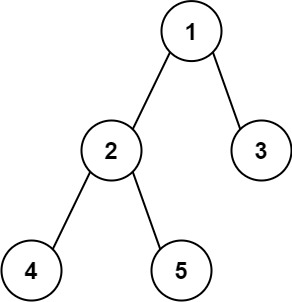| English | 简体中文 |

# 543. Diameter of Binary Tree

## Description

Given the root of a binary tree, return the length of the diameter of the tree.

The diameter of a binary tree is the length of the longest path between any two nodes in a tree. This path may or may not pass through the root.

The length of a path between two nodes is represented by the number of edges between them.

Example 1:Input: root = [1,2,3,4,5]
Output: 3
Explanation: 3 is the length of the path [4,2,1,3] or [5,2,1,3].


Example 2:

Input: root = [1,2]
Output: 1


Constraints:

• The number of nodes in the tree is in the range [1, 104].
• -100 <= Node.val <= 100